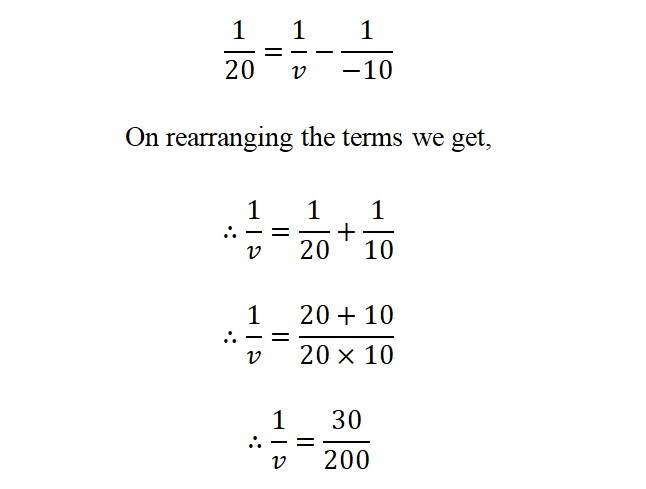# Lens formula

## Lens formula:

If u = object distance, v = image distance and

f = focal length of lens,then the lens formula is given byAccording to sign conventions we need to assume the distance as follows,

Object distance, u= -ve , Image distance, v = -ve/+ve (depends upon the position of image) and Focal length, f= -ve for concave lens/+ve for convex lens.

Let’s consider following example to understand this formula more precisely.

Ex.1) An object is kept in front of converging lens of focal length 20 cm at distance of 10 cm. Find position of image, nature of image.

Solution: Data given ;( using all sign conventions)

Focal length, f= +20 cm

Object distance, u= -10 cm

Image distance=?

Formula:On substituting the above values, we get∴ 1/v = 3/20

∴ v = 20/3

∴ v = 6.67 cm

Positive value of ‘v’ indicates that image is real but inverted.

Magnification by lenses (M):

Magnification is defined as the ratio of height of image (h2) to the height of object (h1) or the ratio of image distance (v) to object distance (u).Ex.2) An object of height 5 cm kept in front of convex lens of focal length 25 cm at distance of 10 cm. Find position of image, nature of image and height of the image.

Solution: Data given ;( using all sign conventions)

Height of object, h1= + 5 cm

Focal length, f= +25 cm

Object distance, u= -10 cm

Image distance=?

Image height=?

Formula:∴ 1/v = 35/200

v = 200/35

∴ v = 5.7 cm

Positive value of ‘v’ indicates that image is real but inverted.

Now to calculate height of image use 2nd formulaHence the height of image obtain here will be 2.85 cm.

(3) The distance of object is 20cm from convex mirror of focal length is 25 cm. Find the position of image?

Given:

F = 15 => convex mirror

Object distance u = -20 cm

Image distance (v) =?

1/f = 1/v + 1/u

1/25 = 1/v + 1/-20

1/25 + 1/20 = 1/v

45/500 = 1/v

V = 500/45 = 11.11

V = 11.1100

The value of  V is +ve so image is virtual.

(4) The radius of curvature is 38 cm. Find the focal length of convex mirror?

Given:

R = 38 cm

Focal length is equal to half of radius of curvature

Focal length = R/2

= 28/2

F = 19 cm

Focal length is 19 cm

(5) Find the position of image if the focal length of convex lens (f) is 8 cm and object is placed 16 cm from lens.

Given:

f = 8 cm

u = – 16 cm

v =?

1/f = 1/v – 1/u

1/8 = 1/v – 1/16

1/v = 1/8 – 1/16

1/v = 1/16

V = 16 cm

∴ The image is placed 16 cm from the principal axis.

Updated: September 24, 2021 — 4:03 pm